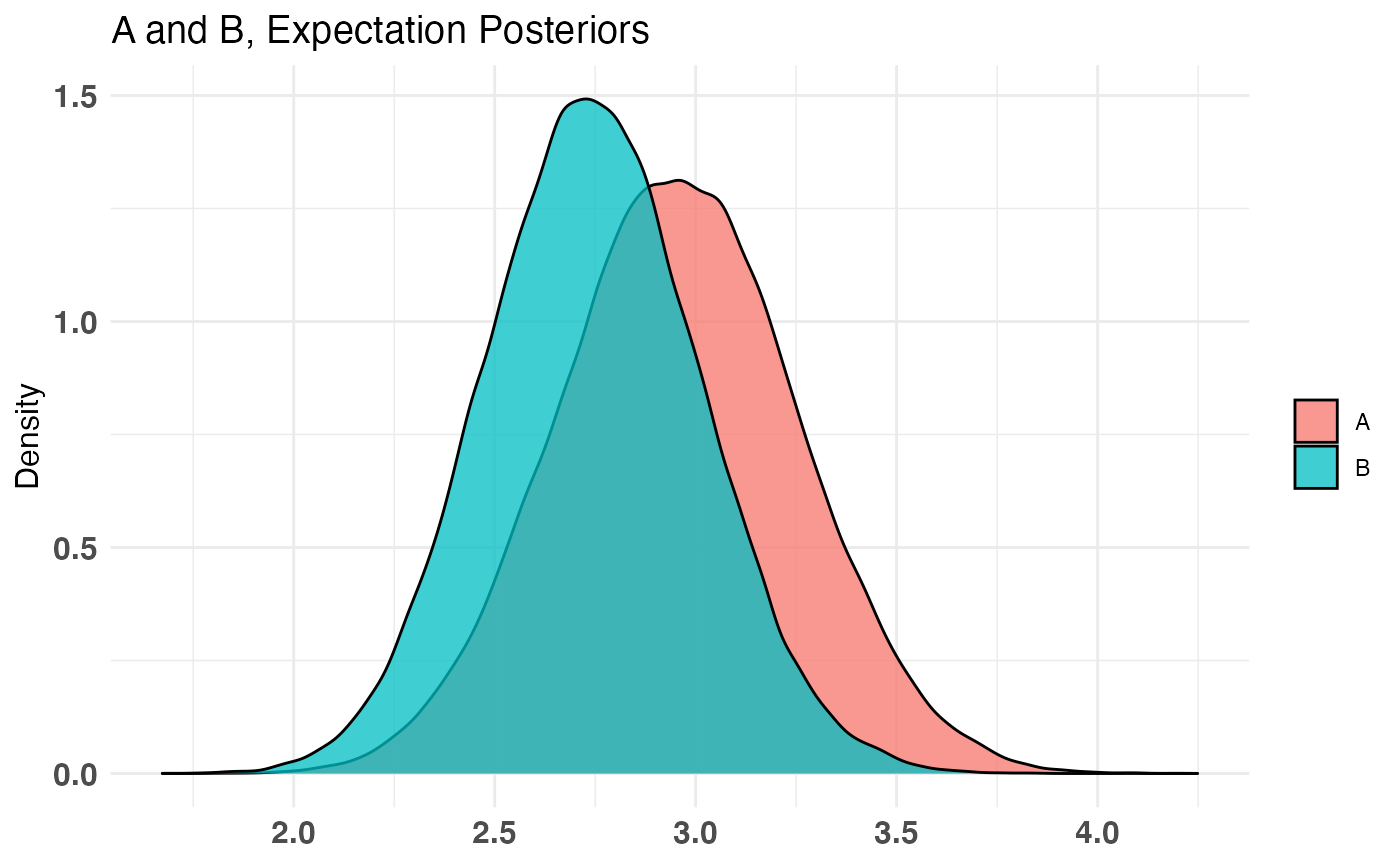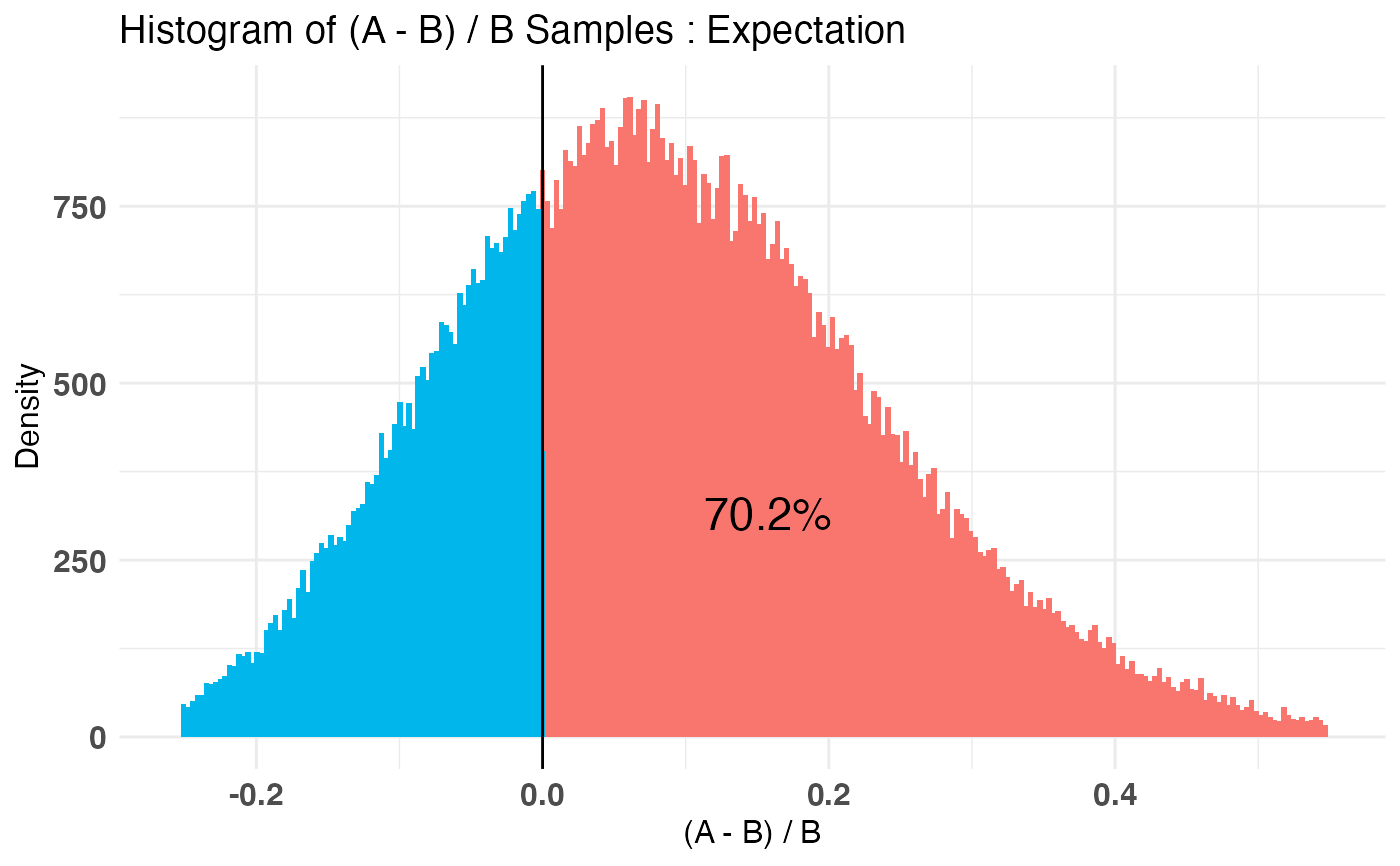This function fits a Bayesian model to your A/B testing sample data. See Details for more information on usage.

bayesTest(A_data, B_data, priors, n_samples = 1e+05,
distribution = c("bernoulli", "normal", "lognormal", "poisson",
"exponential", "uniform", "bernoulliC", "poissonC"))

## Arguments

A_data Vector of collected samples from recipe A Vector of collected samples from recipe B Named vector or named list providing priors as required by the specified distribution: For 'bernoulli' distribution list("alpha" = val1, "beta" = val2) For 'normal' distribution c("mu" = val1, "lambda" = val2, "alpha" = val3, "beta" = val4) For 'lognormal' distribution c("mu" = val1, "lambda" = val2, "alpha" = val3, "beta" = val4) For 'poisson' distribution c("shape" = val1, "rate" = val2) For 'exponential' distribution list("shape" = val1, "rate" = val2) For 'uniform' distribution c("xm" = val1, "alpha" = val2) For 'bernoulliC' distribution: same prior definitions as 'bernoulli' For 'poissonC' distribution: same prior definitions as 'poisson' See plotDistributions or the Note section of this help document for more info. Number of posterior samples to draw. Should be large enough for the distribution to converge. 1e5 is a good rule of thumb. Not used for closed form tests. Distribution of underlying A/B test data.

## Value

A bayesTest object of the appropriate distribution class.

## Details

bayesTest is the main driver function of the bayesAB package. The input takes two vectors of data, corresponding to recipe A and recipe B of an A/B test. Order does not matter, except for interpretability of the final plots and intervals/point estimates. The Bayesian model for each distribution uses conjugate priors which must be specified at the time of invoking the function. Currently, there are eight supported distributions for the underlying data:

• Bernoulli: If your data is well modeled by 1s and 0s, according to a specific probability p of a 1 occurring

• Data must be in a {0, 1} format where 1 corresponds to a 'success' as per the Bernoulli distribution

• Uses a conjugate Beta distribution for the parameter p in the Bernoulli distribution

• alpha and beta must be set for a prior distribution over p

• alpha = 1, beta = 1 can be used as a diffuse or uniform prior

• Normal: If your data is well modeled by the normal distribution, with parameters $$\mu$$, $$\sigma^2$$ controlling mean and variance of the underlying distribution

• Uses a conjugate NormalInverseGamma distribution for the parameters $$\mu$$ and $$\sigma^2$$ in the Normal Distribution.

• mu, lambda, alpha, and beta must be set for prior distributions over $$\mu, \sigma^2$$ in accordance with the parameters of the conjugate prior distributions:

• $$\mu, \sigma^2$$ ~ NormalInverseGamma(mu, lambda, alpha, beta)

• This is a bivariate distribution (commonly used to model mean and variance of the normal distribution). You may want to experiment with both this distribution and the plotNormal and plotInvGamma outputs separately before arriving at a suitable set of priors for the Normal and LogNormal bayesTest

.

• LogNormal: If your data is well modeled by the log-normal distribution, with parameters $$\mu$$, $$\sigma^2$$ as the parameters of the corresponding log-normal distribution (log of data is ~ N($$\mu$$, $$\sigma^2$$))

• The Bayesian model requires same conjugate priors on $$\mu$$, $$\sigma^2$$ as for the Normal Distribution priors

• Note: The $$\mu$$ and $$\sigma^2$$ are not the mean/variance of lognormal numbers themselves but are rather the corresponding parameters of the lognormal distribution. Thus, posteriors for the statistics 'Mean' and 'Variance' are returned alongside 'Mu' and 'Sig_Sq' for interpretability.

• Poisson: If your data is well modeled by the Poisson distribution, with parameter $$\lambda$$ controlling the average number of events per interval.

• Data must be strictly integral or 0.

• Uses a conjugate Gamma distribution for the parameter $$\lambda$$ in the Poisson Distribution

• shape and rate must be set for prior distribution over $$\lambda$$

• Exponential: If your data is well modeled by the Exponential distribution, with parameter $$\lambda$$ controlling the rate of decay.

• Data must be strictly >= 0

• Uses a conjugate Gamma distribution for the parameter $$\lambda$$ in the Exponential Distribution

• shape and rate must be set for prior distribution over $$\lambda$$

• Uniform: If your data is well modeled by the Uniform distribution, with parameter $$\theta$$ controlling the max value.

• For example, estimating max/total inventory size from individually numbered snapshots

• Data must be strictly > 0

• Uses a conjugate Pareto distribution for the parameter $$\theta$$ in the Uniform(0, $$\theta$$) Distribution

• xm and alpha must be set for prior distribution over $$\theta$$

• BernoulliC: Closed form (computational) calculation of the 'bernoulli' bayesTest. Same priors are required.

• PoissonC: Closed form (computational) calculation of the 'poisson' bayesTest. Same priors are required.

## Note

For 'closed form' tests, you do not get a distribution over the posterior, but simply P(A > B) for the parameter in question.

Choosing priors correctly is very important. Please see http://fportman.com/blog/bayesab-0-dot-7-0-plus-a-primer-on-priors/ for a detailed example of choosing priors within bayesAB. Here are some ways to leverage objective/diffuse (assigning equal probability to all values) priors:

• Gamma(eps, eps) ~ Gamma(.00005, .00005) will be effectively diffuse

• InvGamma(eps, eps) ~ InvGamma(.00005, .00005) will be effectively diffuse

• Pareto(eps, eps) ~ Pareto(.005, .005) will be effectively diffuse

Keep in mind that the Prior Plots for bayesTest's run with diffuse priors may not plot correctly as they will not be truncated as they approach infinity. See plot.bayesTest for how to turn off the Prior Plots.

## Examples

A_binom <- rbinom(100, 1, .5)
B_binom <- rbinom(100, 1, .6)

A_norm <- rnorm(100, 6, 1.5)
B_norm <- rnorm(100, 5, 2.5)

AB1 <- bayesTest(A_binom, B_binom,
priors = c('alpha' = 1, 'beta' = 1),
distribution = 'bernoulli')
AB2 <- bayesTest(A_norm, B_norm,
priors = c('mu' = 5, 'lambda' = 1, 'alpha' = 3, 'beta' = 1),
distribution = 'normal')

print(AB1)#> --------------------------------------------
#> Distribution used: bernoulli
#> --------------------------------------------
#> Using data with the following properties:
#>            A   B
#> Min.    0.00 0.0
#> 1st Qu. 0.00 0.0
#> Median  0.00 1.0
#> Mean    0.41 0.6
#> 3rd Qu. 1.00 1.0
#> Max.    1.00 1.0
#> --------------------------------------------
#> Conjugate Prior Distribution: Beta
#> Conjugate Prior Parameters:
#> $alpha #>  1 #> #>$beta
#>  1
#>
#> --------------------------------------------
#> Calculated posteriors for the following parameters:
#> Probability
#> --------------------------------------------
#> Monte Carlo samples generated per posterior:
#>  1e+05summary(AB1)#> Quantiles of posteriors for A and B:
#>
#> $Probability #>$Probability$A #> 0% 25% 50% 75% 100% #> 0.2303915 0.3787600 0.4113576 0.4443736 0.6189084 #> #>$Probability$B #> 0% 25% 50% 75% 100% #> 0.3865806 0.5652217 0.5984563 0.6313930 0.7917407 #> #> #> -------------------------------------------- #> #> P(A > B) by (0)%: #> #>$Probability
#>  0.00404
#>
#> --------------------------------------------
#>
#> Credible Interval on (A - B) / B for interval length(s) (0.9) :
#>
#> $Probability #> 5% 95% #> -0.4602176 -0.1331493 #> #> -------------------------------------------- #> #> Posterior Expected Loss for choosing B over A: #> #>$Probability
#>  0.4721247
#> plot(AB1)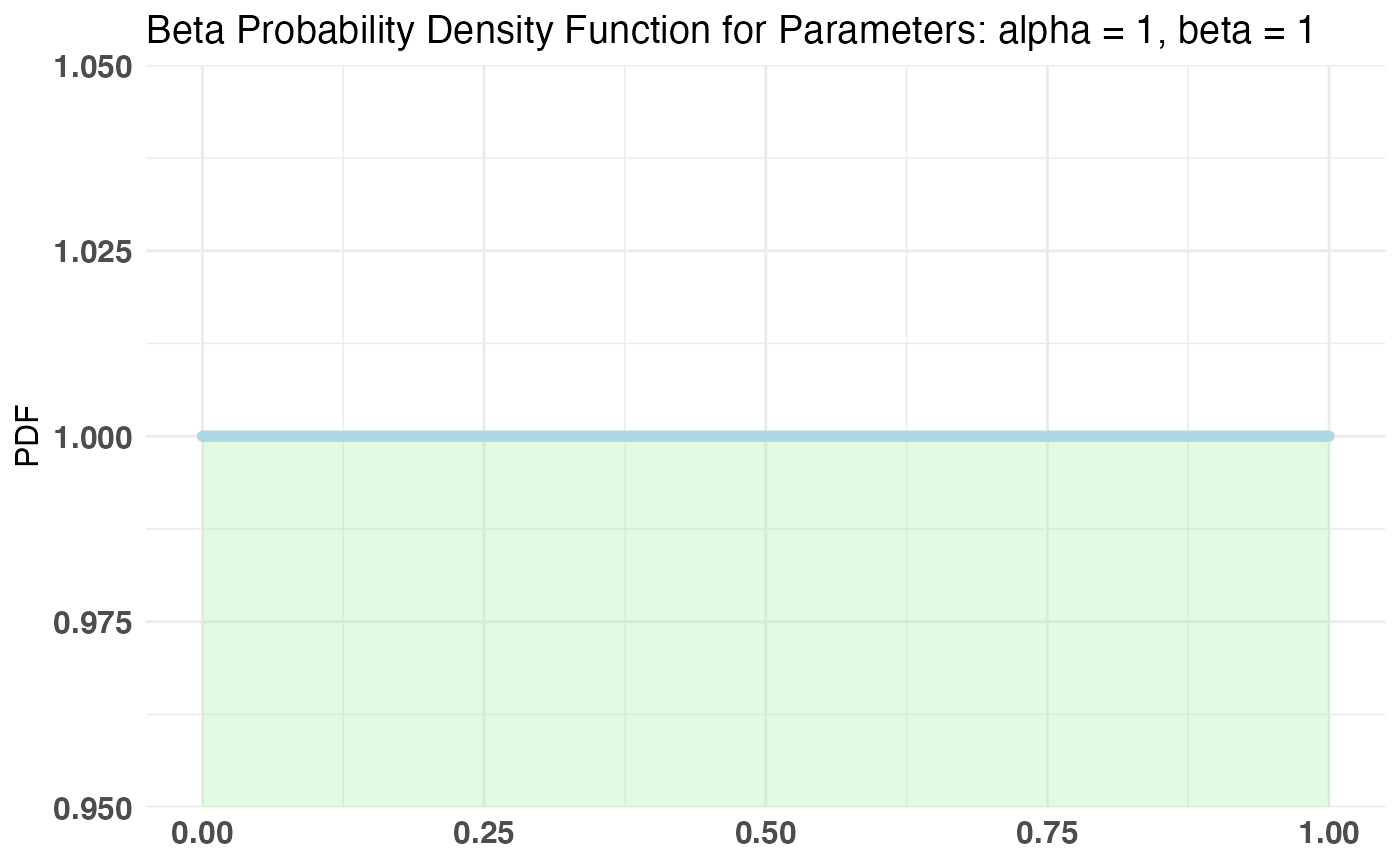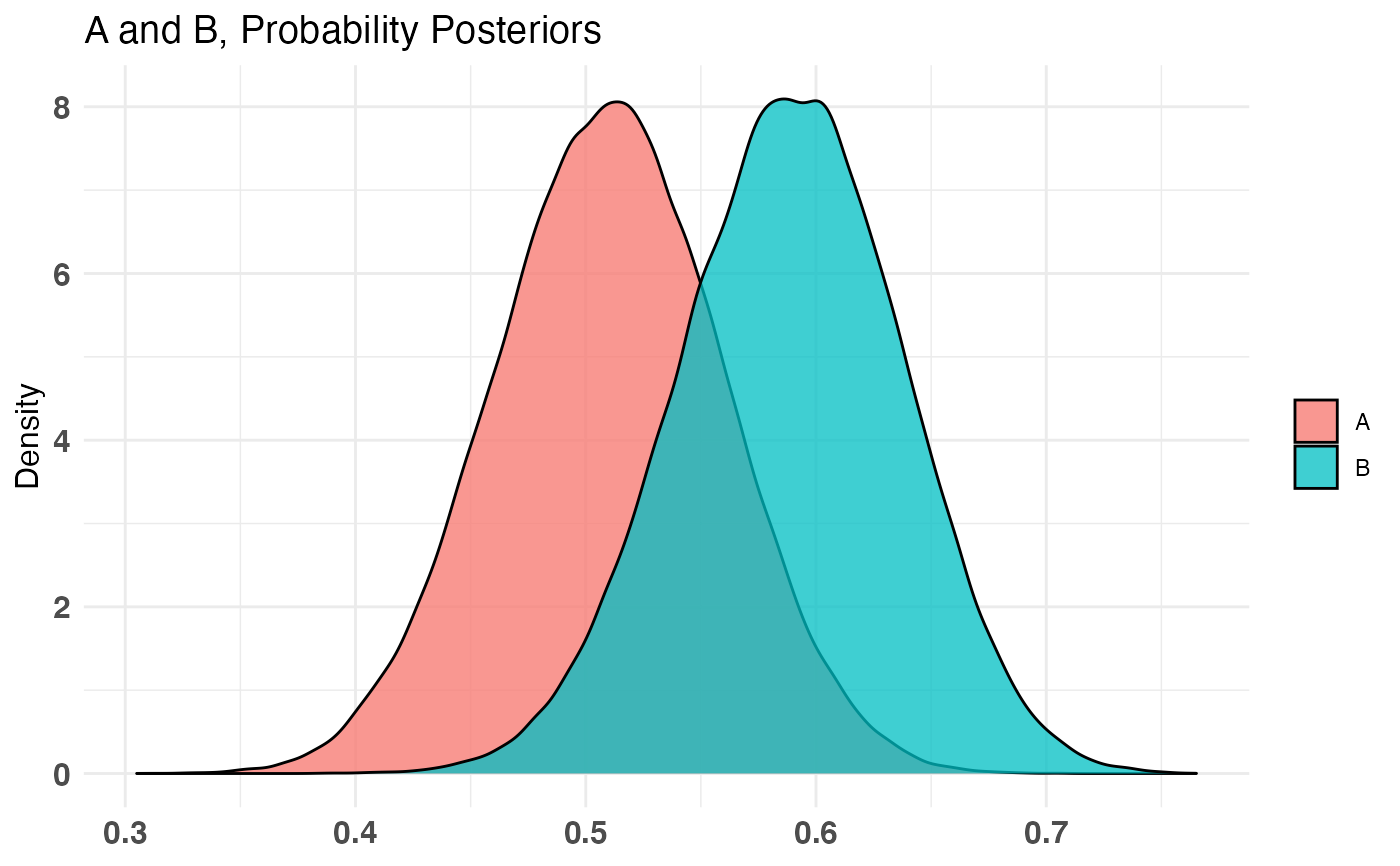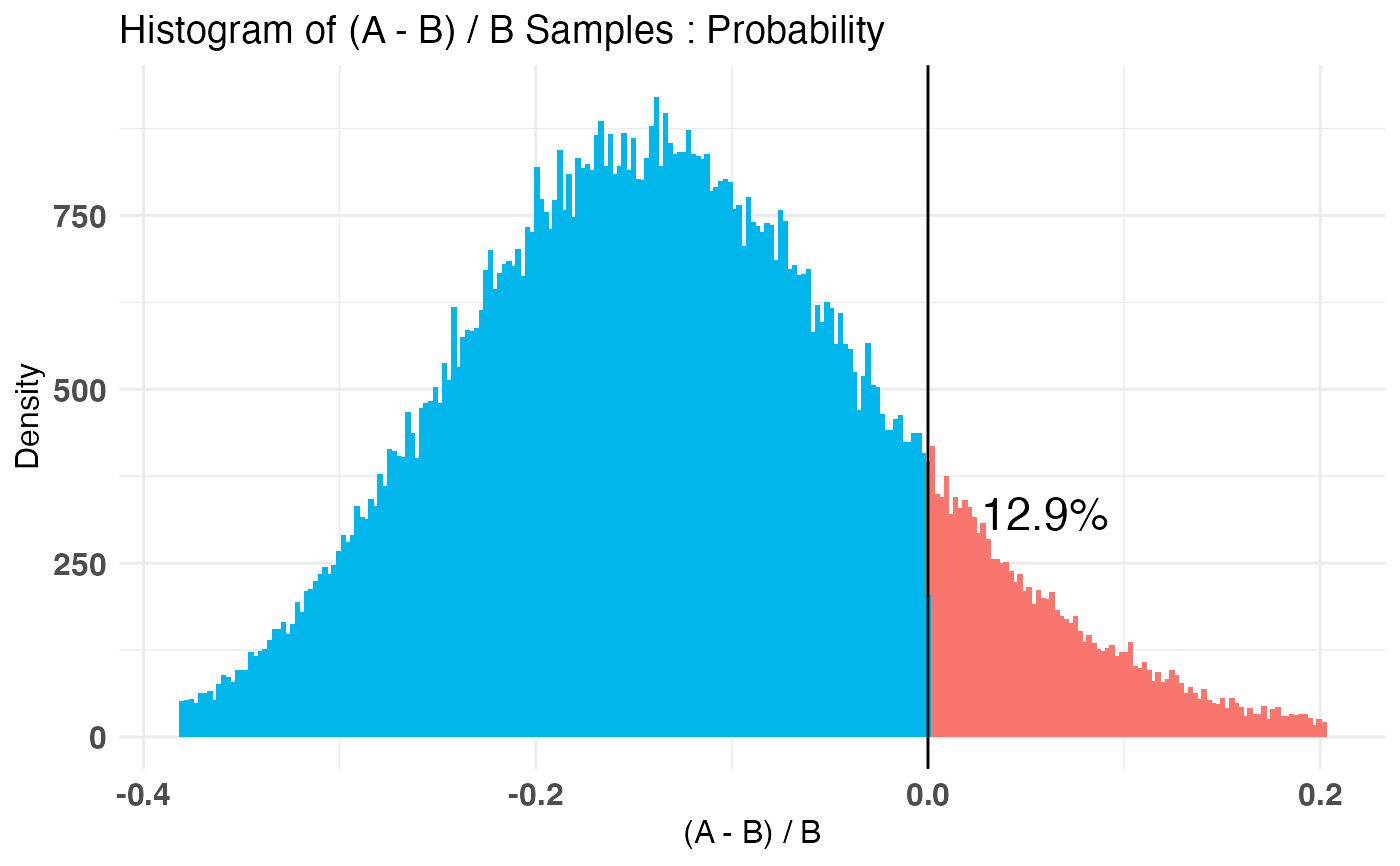summary(AB2)#> Quantiles of posteriors for A and B:
#>
#> $Mu #>$Mu$A #> 0% 25% 50% 75% 100% #> 5.323064 5.937443 6.038791 6.140953 6.745988 #> #>$Mu$B #> 0% 25% 50% 75% 100% #> 3.672163 4.737485 4.914194 5.090027 6.041934 #> #> #>$Sig_Sq
#> $Sig_Sq$A
#>       0%      25%      50%      75%     100%
#> 1.364615 2.115471 2.316705 2.546459 4.298706
#>
#> $Sig_Sq$B
#>        0%       25%       50%       75%      100%
#>  3.494541  6.292978  6.896448  7.572679 15.081372
#>
#>
#> --------------------------------------------
#>
#> P(A > B) by (0, 0)%:
#>
#> $Mu #>  0.99983 #> #>$Sig_Sq
#>  0
#>
#> --------------------------------------------
#>
#> Credible Interval on (A - B) / B for interval length(s) (0.9, 0.9) :
#>
#> $Mu #> 5% 95% #> 0.1181279 0.3581798 #> #>$Sig_Sq
#>         5%        95%
#> -0.7564991 -0.5370850
#>
#> --------------------------------------------
#>
#> Posterior Expected Loss for choosing B over A:
#>
#> $Mu #>  2.544927e-06 #> #>$Sig_Sq
#>  2.032421
#>
# Create a new variable that is the probability multiiplied
# by the normally distributed variable (expected value of something)
AB3 <- combine(AB1, AB2, f = *, params = c('Probability', 'Mu'), newName = 'Expectation')

print(AB3)#> --------------------------------------------
#> Distribution used: combined
#> --------------------------------------------
#> Using data with the following properties:
#>            A         A   B         B
#> Min.    0.00  3.132821 0.0 -3.377552
#> 1st Qu. 0.00  4.895238 0.0  3.250953
#> Median  0.00  5.898531 1.0  4.924260
#> Mean    0.41  6.049633 0.6  4.912154
#> 3rd Qu. 1.00  6.936479 1.0  6.734556
#> Max.    1.00 10.323493 1.0 11.265252
#> --------------------------------------------
#> Conjugate Prior Distribution:
#> Conjugate Prior Parameters:
#>  "Combined distributions have no priors. Inspect each element separately for details."
#> --------------------------------------------
#> Calculated posteriors for the following parameters:
#> Expectation
#> --------------------------------------------
#> Monte Carlo samples generated per posterior:
#>  1e+05summary(AB3)#> Quantiles of posteriors for A and B:
#>
#> $Expectation #>$Expectation$A #> 0% 25% 50% 75% 100% #> 1.364129 2.282603 2.483074 2.686978 3.765758 #> #>$Expectation$B #> 0% 25% 50% 75% 100% #> 1.785842 2.742598 2.934746 3.128652 4.285103 #> #> #> -------------------------------------------- #> #> P(A > B) by (0)%: #> #>$Expectation
#>  0.13842
#>
#> --------------------------------------------
#>
#> Credible Interval on (A - B) / B for interval length(s) (0.9) :
#>
#> $Expectation #> 5% 95% #> -0.34810418 0.08933727 #> #> -------------------------------------------- #> #> Posterior Expected Loss for choosing B over A: #> #>$Expectation
#>  0.2084638
#> plot(AB3)